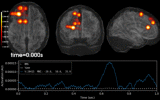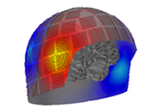# mne.stc_near_sensors¶

mne.stc_near_sensors(evoked, trans, subject, distance=0.01, mode='sum', project=True, subjects_dir=None, src=None, verbose=None)[source]

Create a STC from ECoG, sEEG and DBS sensor data.

Parameters
evokedinstance of `Evoked`

The evoked data. Must contain ECoG, sEEG or DBS channels.

trans`str` | `dict` | instance of `Transform` | `None`

If str, the path to the head<->MRI transform `*-trans.fif` file produced during coregistration. Can also be `'fsaverage'` to use the built-in fsaverage transformation. If trans is None, an identity matrix is assumed.

Changed in version 0.19: Support for ‘fsaverage’ argument.

subject`str`

The subject name.

distance`float`

Distance (m) defining the activation “ball” of the sensor.

mode`str`

Can be “sum” to do a linear sum of weights, “nearest” to use only the weight of the nearest sensor, or “single” to do a distance-weight of the nearest sensor. Default is “sum”. See Notes.

projectbool

If True, project the electrodes to the nearest `'pial` surface vertex before computing distances. Only used when doing a surface projection.

subjects_dir

The path to the FreeSurfer subjects reconstructions. It corresponds to FreeSurfer environment variable `SUBJECTS_DIR`.

srcinstance of `SourceSpaces`

The source space.

Warning

If a surface source space is used, make sure that `surf='pial'` was used during construction.

verbose

If not None, override default verbose level (see `mne.verbose()` and Logging documentation for more). If used, it should be passed as a keyword-argument only.

Returns
stcinstance of `SourceEstimate`

The surface source estimate. If src is None, a surface source estimate will be produced, and the number of vertices will equal the number of pial-surface vertices that were close enough to the sensors to take on a non-zero volue. If src is not None, a surface, volume, or mixed source estimate will be produced (depending on the kind of source space passed) and the vertices will match those of src (i.e., there may be me many all-zero values in stc.data).

Notes

For surface projections, this function projects the ECoG sensors to the pial surface (if `project`), then the activation at each pial surface vertex is given by the mode:

• `'sum'`

Activation is the sum across each sensor weighted by the fractional `distance` from each sensor. A sensor with zero distance gets weight 1 and a sensor at `distance` meters away (or larger) gets weight 0. If `distance` is less than the distance between any two electrodes, this will be the same as `'nearest'`.

• `'single'`

Same as `'sum'` except that only the nearest electrode is used, rather than summing across electrodes within the `distance` radius. As `'nearest'` for vertices with distance zero to the projected sensor.

• `'nearest'`

The value is given by the value of the nearest sensor, up to a `distance` (beyond which it is zero).

If creating a Volume STC, `src` must be passed in, and this function will project sEEG and DBS sensors to nearby surrounding vertices. Then the activation at each volume vertex is given by the mode in the same way as ECoG surface projections.

New in version 0.22.

## Examples using `mne.stc_near_sensors`¶